• 在C语言中，函数的参数和返回值的传递方式有两种：值传递和指针传递值传递和指针传递初学者总会有一种朦胧的感觉，所以建议把指针传递的概念摸透，才能熟练应用。 值传递示例：x其实是n的一份临时拷贝，所以并...
在C语言中，函数的参数和返回值的传递方式有两种：值传递和指针传递。
值传递和指针传递初学者总会有一种朦胧的感觉，所以建议把指针传递的概念摸透，才能熟练应用。

值传递示例：x其实是n的一份临时拷贝，所以并不会改变n的值。

#include <stdio.h>
#include <windows.h>
void Fun(int x)
{
x = 1;
}

int main()
{
int n = 2;
Fun(n);
printf("%d\n",n);
system("pause");
return 0;
}

程序结果：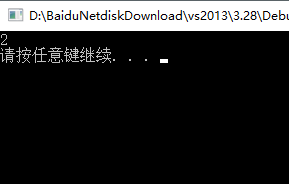指针传递：这个地方可以理解为函数在被调用的时候n把地址给了x，它们都是同一个内存地址，所以x改变就随着变化。#include <stdio.h>
#include <windows.h>
void Fun(int *x)
{
*x = 1;
}

int main()
{
int n = 2;
Fun(&n);
printf("%d\n",n);
system("pause");
return 0;
}
程序结果：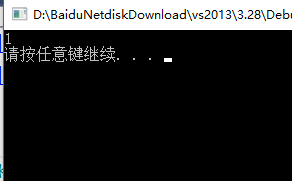展开全文• C语言中 值传递和指针传递实例详解 在C语言中，函数的参数和返回值的传递方式有两种：值传递和指针传递值传递和指针传递初学者总会有一种朦胧的感觉，所以建议把指针传递的概念摸透，才能熟练应用。 值传递示例...
• Golang值传递和指针传递 package main import ( "fmt" ) func swap1(x, y, p *int) { if *x > *y { *x, *y = *y, *x } *p = *x * *y } func swap2(x, y int) (int, int, int) { if x >...
Golang值传递和指针传递
package main

import (
"fmt"
)

func swap1(x, y, p *int) {
if *x > *y {
*x, *y = *y, *x
}
*p = *x * *y
}

func swap2(x, y int) (int, int, int) {
if x > y {
x, y = y, x
}
return x, y, x * y
}

func main() {
i := 9
j := 5
product := 0
swap1(&i, &j, &product)
fmt.Println(i, j, product)    //5 9 45

a := 64
b := 23
a, b, p := swap2(a, b)
fmt.Println(a, b, p)   //23 64 1472
}

转载于:https://www.cnblogs.com/tomtellyou/p/10433535.html
展开全文• 一、值传递 将要传递的值作为副本传递。传递过程中被调函数的形参作为被调函数的局部变量处理，在内存堆栈中开辟新空间以存放主调函数传过来的实参值，从而成为实参...三、指针传递和引用传递的区别 指针和引用都是
一、值传递
将要传递的值作为副本传递。传递过程中被调函数的形参作为被调函数的局部变量处理，在内存堆栈中开辟新空间以存放主调函数传过来的实参值，从而成为实参的副本

二、引用于值传递的区别
1、引用传递传递的是引用对象的内存地址，被调函数的形参存放主调函数传递进来实参变量的地址，即通过堆栈中存放地址访问主调函数中的实参变量

三、指针传递和引用传递的区别
指针和引用都是关于地址的概念，指针指向一块地址，内容为所指内存的地址。二引用是某块内存的别名。
引用只能在定义时初始化一次，之后不可改变；指针可变。
引用不可为空，指针可以。
“sizeof引用”得到所指向变量的大小，“sizeof指针”得到指针本身（指向对象地址的）大小。
展开全文• java–关于值传参和引用传参 java作为面向对象的编程语言，其语法其实和C差不多 ...c值传递和指针传递： #include void swap(int a,int b){ int temp = a; a = b; b = temp; printf("2:a = %d,b = %d
java–关于值传参和引用传参

java作为面向对象的编程语言，其语法其实和C差不多
相信作为编程初学者，对于写一个交换两个变量的值的函数应该遇到过该问题

c值传递和指针传递：

#include<stdio.h>
void swap(int a,int b){
int temp = a;
a = b;
b = temp;
printf("2:a = %d,b = %d\n",a,b);
}
int main(){
int a = 1;
int b = 2;
printf("1:a = %d,b = %d\n",a,b);
swap(a,b);
printf("3:a = %d,b = %d\n",a,b);
return 0 ;
}

输出结果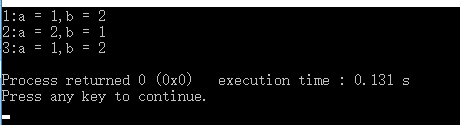本来使用交换函数swapF()后第三行的输出应该是和第二行的输出一样的，但现在却是和预期输出不一样，那么，很多初学者就很疑惑了，为什么我交换了a,b的值，但是输出时还是不一样呢？这里要提到C的指针（通俗理解就是内存地址），由于主函数中的a,b变量所在的内存地址存的值是1和2，传递参数的时候传递的是a,b的值，而不是传递的a,b变量所在的内存地址，所以我们可以看下修改后的代码

#include<stdio.h>
void swap(int *a,int *b){
int temp = *a;
*a = *b;
*b = temp;
printf("3:a = %d,b = %d\n",a,b);
printf("4:a = %d,b = %d\n",*a,*b);
}
int main(){
int a = 1;
int b = 2;
printf("1:a = %d,b = %d\n",a,b);
printf("2:a = %d,b = %d\n",&a,&b);
swap(&a,&b);
printf("5:a = %d,b = %d\n",a,b);
return 0 ;
}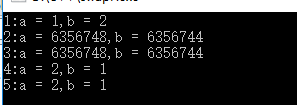现在看这五行输出 最后一行输出已经达到我们预期的输出，我们改动的地方在这里：由于直接传递变量的值不能交换两个变量的值，所以我们第二次传递的是两个变量的所在的内存地址，在新的swapF()里，取变量地址锁存的值进行交换，这个时候a变量所在的内存地址的值已经变为原来b变量所存的值了，可以看第2，3行，a,b变量所在的内存地址是没有变的,但看1,5行，a,b变量所存的值这个时候已经在swapF()函数中已a变

再来看java的值传递和引用传递

看了上面的例子介绍，我相信初学者们差不多应该知道java的值传递和引用传递是怎么回事了，没错，值传递就是和上面的传递变量值，引用传递就是上面的地址传值
可以看看代码，很好理解：

package com.brucewee.test;
public class Main
{
public static void main(String[] args)
{
//引用类型传参
Person p = new Person();
p.setNameAndAge("张三", 20);
System.out.println("name:"+p.getName()+"-age:"+p.getAge());
point(p);
System.out.println("name:"+p.getName()+"-age:"+p.getAge());
//值类型传参
int a = 10;
value(a);
System.out.println("a = "+a);

}
public static void value(int a){
a = 20;
System.out.println("a = "+a);
}
public static void point(Person p){
p.setNameAndAge("王五", 18);
System.out.println("name:"+p.getName()+"-age:"+p.getAge());
}
}
class Person{
private String name;
private int age;
public String getName(){
return this.name;
}
public int getAge(){
return this.age;
}
public void setNameAndAge(String name,int age){
this.name;
= name;
this.age = age;
}
}

输出结果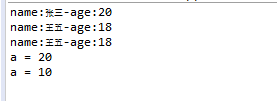这里要先说下，在java中基础类型是值传递，对象类型是引用传递，
我新建了一个Person类 里面有成员name和age,还有他们的get,set方法
value()函数展示值传递，point()函数展示引用传递
对比上面的两个swapF()函数，value()就相当于第一个swapF()函数,point()就相当于第二个swapF()函数
value()函数传递的参数a是传递的int型变量a的值,而point()函数传递的参数p是Person对象（也就是对象p所分配的内存地址）
展开全文• a= 1def change_integer(a):a=a+1return aprint change_integer(a)print ab= [1, 2, 3]def change_list(b):b= b+1return bprint change_list(b)print b第一个例子，我们将一个整数变量传递给函数，函数对它...
• 指针传递参数本质上是值传递的方式，所传递的是一个地址值。值传递过程中，被调函数的形式参数被作为被调函数的局部变量处理，即在栈中开辟了内存空间以存放由主调函数放进来的实参的值，从而成为了实参的一个副本。...
• a= 1def change_integer(a):a=a+1return a print change_integer(a)print a b= [1, 2, 3]def change_list(b):b= b+1return bprint change_list(b)print b第一个例子，我们将一个整数变量传递给函...
• 以前只是知道值传递不能改变实参，忘了是为啥，上网查了下，主要就两点；函数的形参函数内声明的变量都是该函数的局部变量，而局部变量在函数执行完就释放了，其次 ，形参只是实参的一份拷贝，二者具有不同的地址...
• a= 1def change_integer(a):a=a+1return aprint change_integer(a)print ab= [1, 2, 3]def change_list(b):b= b+1return bprint change_list(b)print b第一个例子，我们将一个整数变量传递给函数，函数对它...
• 值传递就是最普通的传递方式，比如函数定义为fun(int a)，在调用的地方有int x=6， 使用fun(x)就可以了。这种方式在fun(int a)函数内部的对a的修改 不能 导致外部x的变化。 指针传递其实也就是地址传递，函数定义为...
• 函数的值传递 main中初始化整型变量 int a,b; 定义一个函数void swap(int a,int b) main程序中调用函数swap(a,b) 此时swap函数接收到的是整型a,b而不是变量a,b 当我们在swap中执行赋值时,改变的是函数内a,b变量的值...java c语言
• package main import "fmt" func swap1(a,b int) (int,int){//通过值，进行交换 return b,a } func swap2(a,b *int){//交换指针地址 ... //a,b=swap1(a,b)//值传递 swap2(&amp;...
• 函数中的nmain中的存储n的内存地址是不一样的，但是都是存储了n的(n是指针)。 3 . A 函数任何传递指针、非引用的参数，都会在 A 函数的函数栈内开辟空间复制这个，如果是数组，都会复制该数组在该函数，...c golang 引用传递
• 在把数组作为参数传递给函数时，有值传递(by value)地址传递(by reference)两种方式。在值传递方式中，在说明定义函数时，要在数组参数的尾部加上一对方括号([])，调用函数时只需将数组的地址(即数组名)传递给......